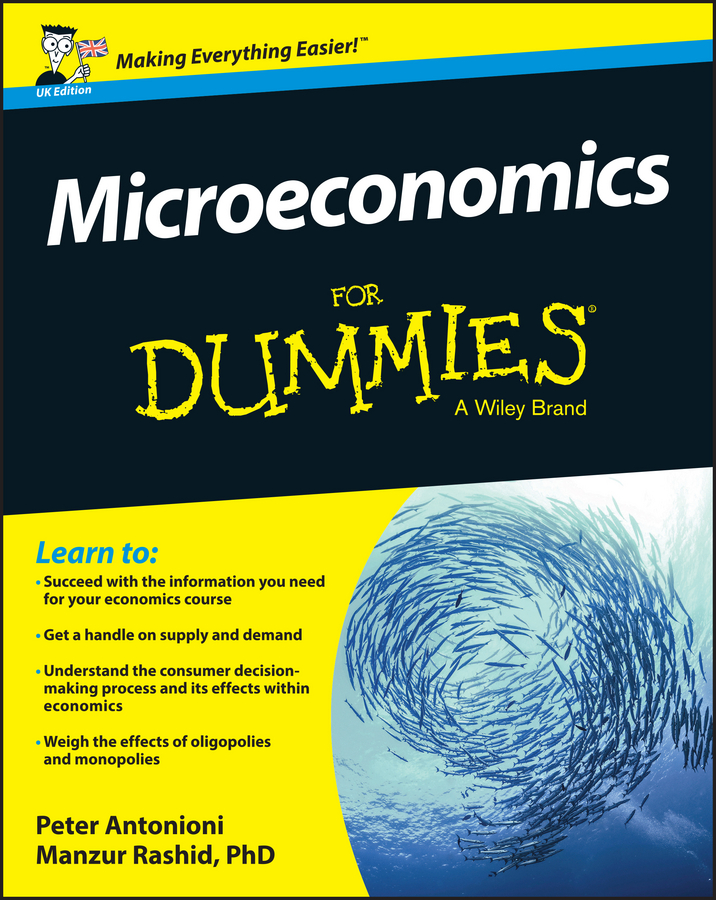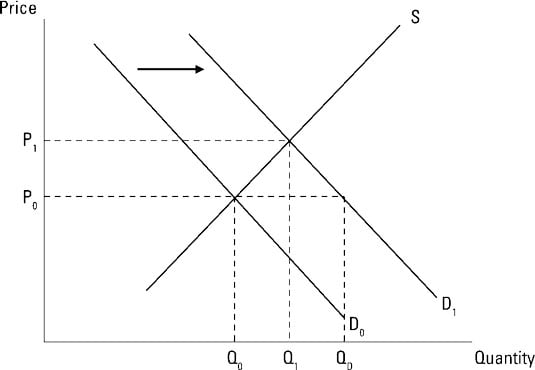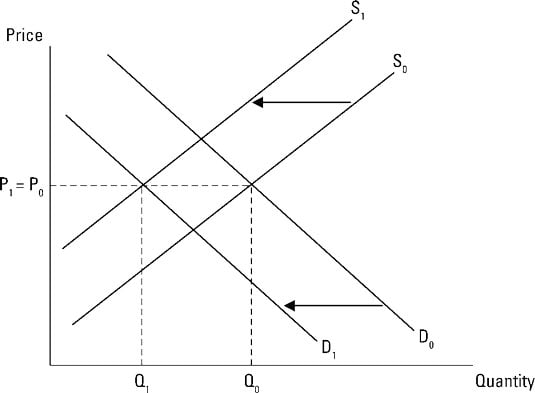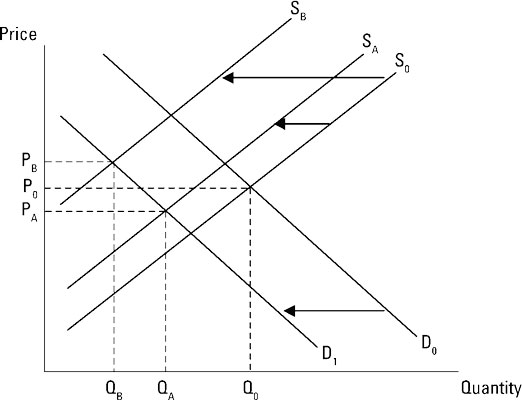##### Microeconomics For Dummies - UKEconomic markets tend toward equilibrium, the price and quantity that correspond to the point where supply and demand intersect. But equilibrium itself can change.

Because equilibrium corresponds to the point where the demand and supply curves intersect, anything that shifts the demand or supply curves establishes a new equilibrium.

The illustration shows what happens when demand increases. Originally, the market was in equilibrium at price P0 and quantity Q0. If demand increases, the demand curve shifts to the right from D0 to D1. The quantity demanded associated with the price P0 is now QD.

Because this is greater than the quantity producers are providing (still Q0 as determined off the supply curve), a shortage exists. The market moves from the original equilibrium price P0 to the new equilibrium price P1 and from the original equilibrium quantity Q0 to the new equilibrium quantity, Q1.The impact of an increase in supply is illustrated below. Originally, the equilibrium price and quantity are P0 and Q0, respectively. An increase in supply shifts the supply curve to the right from S0 to S1. The supply increase immediately creates a surplus because at P0, the new quantity supplied QS is greater than the quantity demanded, which is still at Q0.

Because there is a surplus, the good’s price falls from P0 to the new equilibrium price P1, and the quantity demanded and quantity supplied move to the new equilibrium quantity Q1, which is greater than the original equilibrium quantity Q0.

There are instances where both demand and supply shift at the same time, and this makes determining the changes in equilibrium price and quantity more difficult.When both demand and supply shift simultaneously, the change in only one equilibrium characteristic — price or quantity — can be definitely determined.

The illustration below shows a simultaneous decrease in both demand and supply — the demand curve shifts left from D0 to D1, and the supply curve shifts left from S0 to S1. The original equilibrium price and quantity are P0 and Q0, corresponding to the intersection of the original demand and supply curves.

Given the shifts to D1 and S1, the equilibrium quantity decreases from Q0 to Q1 while the equilibrium price has not changed — P0 = P1. But note that in this illustration, the demand and supply curves shift by the same amount.In the next illustration, two decreases in supply are illustrated along with the decrease in demand. The first decrease in supply is a relatively small one, from S0 to SA. The new equilibrium quantity decreases from Q0 to QA, and the equilibrium price also decreases from P0 to PA.

The second decrease in supply is a relatively large one, from S0 to SB. In this case, the new equilibrium quantity still decreases, now from Q0 to QB. But note what happens to equilibrium price: It increases from P0 to PB. Given the decrease in demand, a small decrease in supply results in a lower equilibrium price, while a large decrease in supply results in a higher equilibrium price.These two illustrations show that when both demand and supply simultaneously decrease, equilibrium quantity always decreases, but equilibrium price can increase, decrease, or remain the same. So, only one equilibrium characteristic — equilibrium quantity — can be definitely determined.

The crucial thing to note is that no matter what happens to supply and demand, the market always adjusts to its equilibrium point.